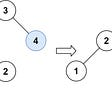# Opening

Given a non-negative integer `num`, return the number of steps to reduce it to zero. If the current number is even, you have to divide it by 2, otherwise, you have to subtract 1 from it.

Example 1:

`Input: num = 14Output: 6Explanation: Step 1) 14 is even; divide by 2 and obtain 7. Step 2) 7 is odd; subtract 1 and obtain 6.Step 3) 6 is even; divide by 2 and obtain 3. Step 4) 3 is odd; subtract 1 and obtain 2. Step 5) 2 is even; divide by 2 and obtain 1. Step 6) 1 is odd; subtract 1 and obtain 0.`

Example 2:

`Input: num = 8Output: 4Explanation: Step 1) 8 is even; divide by 2 and obtain 4. Step 2) 4 is even; divide by 2 and obtain 2. Step 3) 2 is even; divide by 2 and obtain 1. Step 4) 1 is odd; subtract 1 and obtain 0.`

Example 3:

`Input: num = 123Output: 12`

Constraints:

• `0 <= num <= 10^6`

# Solution

From my experience, easy problems on LeetCode are problems that tackle specific concepts. Medium problems tackle advanced concepts and hard problems put everything together.

This is one of those problems that go in between easy and medium. Easy because conceptually, it’s just a while loop. Medium because if this was an interview question, your interviewer expects to see a binary solution.

Let’s start off easy. The pseudocode for easy:

`given a number, start a counterif the current number is even, divide by 2else subtract from 1return counter once the number reaches to 0`

Let’s put this in Python:

`class Solution:    def numberOfSteps (self, num: int) -> int:        count = 0        result = -1        while (result != 0):            if (num % 2 == 0):                num = num / 2            else:                num = num - 1            count = count + 1            result = num        return count`

First start the count at 0 and result at some non-zero. -1 makes the most sense. While the result is not 0, if the current number is even, divide by 2 or else subtract by 1.

You could do away with result and just use the num itself. This means while num is greater than 0, run the rest. Anyway, as you divide or subtract, you are incrementing each step and setting num to result.

Once result hits 0, you return the count.

This works. It’s not even bad, it’s running at O(log(n)). This is because we are effectively halving whenever it’s an even number. And it will always be an even number if we subtract 1 from an odd number.

If you’re nervous, you could just use this solution and hope they understand that you’ll give a more elegant solution in the real world. Especially if you shake like a leaf, like me.

During an interview, it’s probably best to just go straight to the binary solution. It’ll prevent you from wasting time on a loop solution if you aren’t going to give it in the first place.

If you notice something, 0001 is an odd number. 0000 is an even number. It doesn’t matter what the rest of the binary is. Let’s use an example to see what I mean.

Let’s use 51. This is 110011 in binary or 11011_2. I’ll just assume you can intuit that if I use only 0s and 1s, I’m writing binaries.

Anyway, what’s 50? 110010
25? 011001
24? 011000
12? 001100
6? 000110
3? 000011
2? 000010
1? 000001
0? 000000

You’ll notice how the bits are shifting to the right. It took one step to remove 0s and two steps to remove 1s.

More specifically, how? When it’s 0, you are halving, which means 1 bit shift. That’s one step. When it’s 1, you subtract by 1, before you do the halving, which is two steps.

So you multiply 0s by 1 and 1s by 2 and subtract the entire resulting number by 1 since the last bit always takes only one to remove.

So, using 110011, we could just count and create a formula for this. (2 * 1) + (4 * 2) - 1 = 9

When you write the code, you would just need to convert the decimal to binary and count the 0s and 1s. Then you multiply accordingly and then finally subtract by one.

There’s a couple of approaches to this and really depends on how drawn out this has to be. For example, instead of writing the bin() function, you could roll your own.

If you want something a little more Pythonic, it could look something like:

`class Solution:    def numberOfSteps (self, num: int) -> int:        binary = bin(num)        binary = binary[2:]        ones = binary.count("1")        zeroes = binary.count("0")        total = (ones * 2) + (zeroes * 1) - 1        return total`

It’s rather straightforward, you convert num into binary with bin(). Then you slice the string to get rid of the “2b.”

Count the ones and zeroes. Add the counts and subtract by one. Return the final value. You could, of course, continue to make it more Pythonic but this works as an explanation.

Good luck!

--

--

--

I write just in case death knocks

Love podcasts or audiobooks? Learn on the go with our new app.

## What does fit in a low resources namespace? 3rd part. Inlets## Common Python Anti-Patterns to watch out for## A pragmatic architecture — Part 1## Our vision## Why You Should Never Consent to a Coding Test in an Interview## Winja CTF #5 Writeup## Cassy

I write just in case death knocks

## Meta / Amazon / Google / Microsoft| Trim a Binary Search Tree | LeetCode-669: Medium | JavaScript…